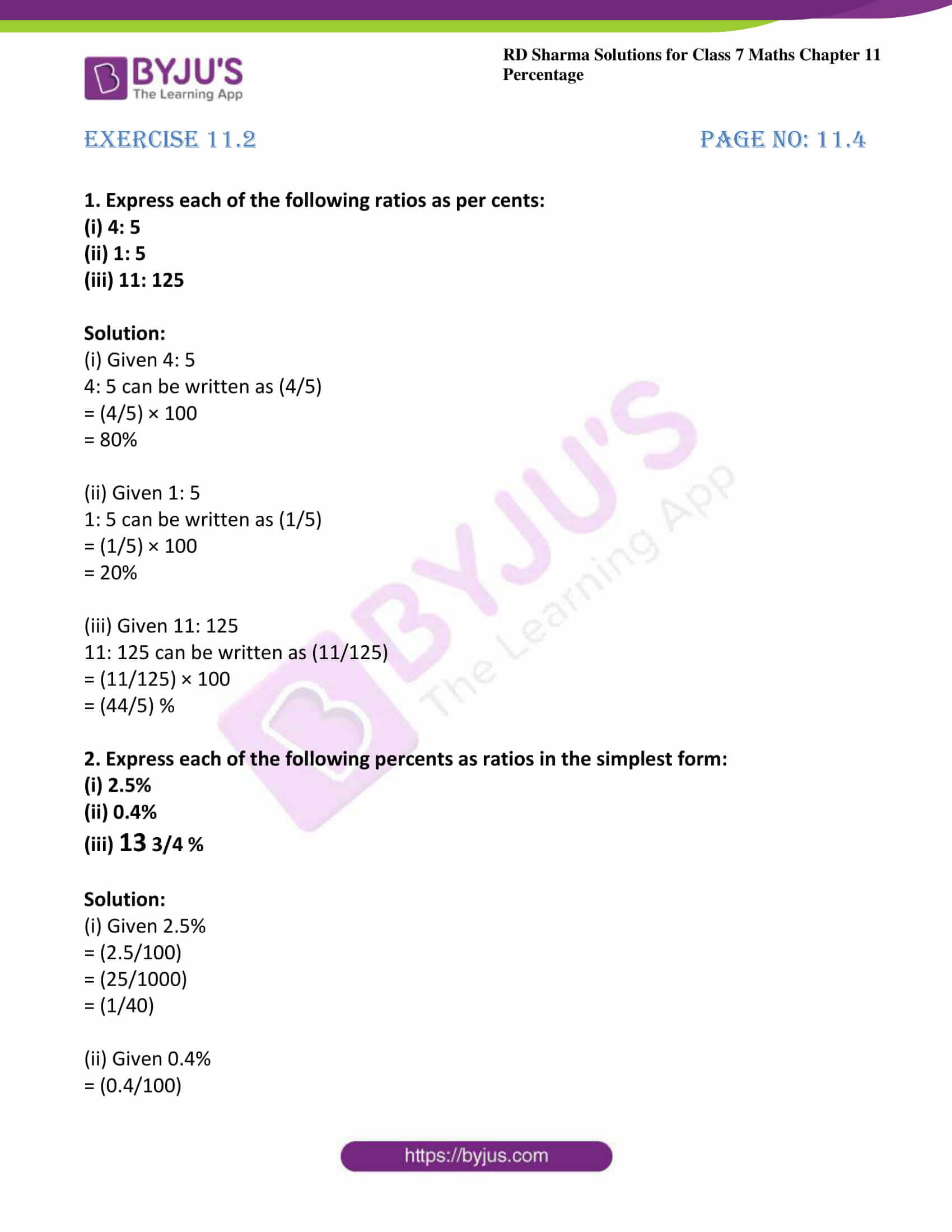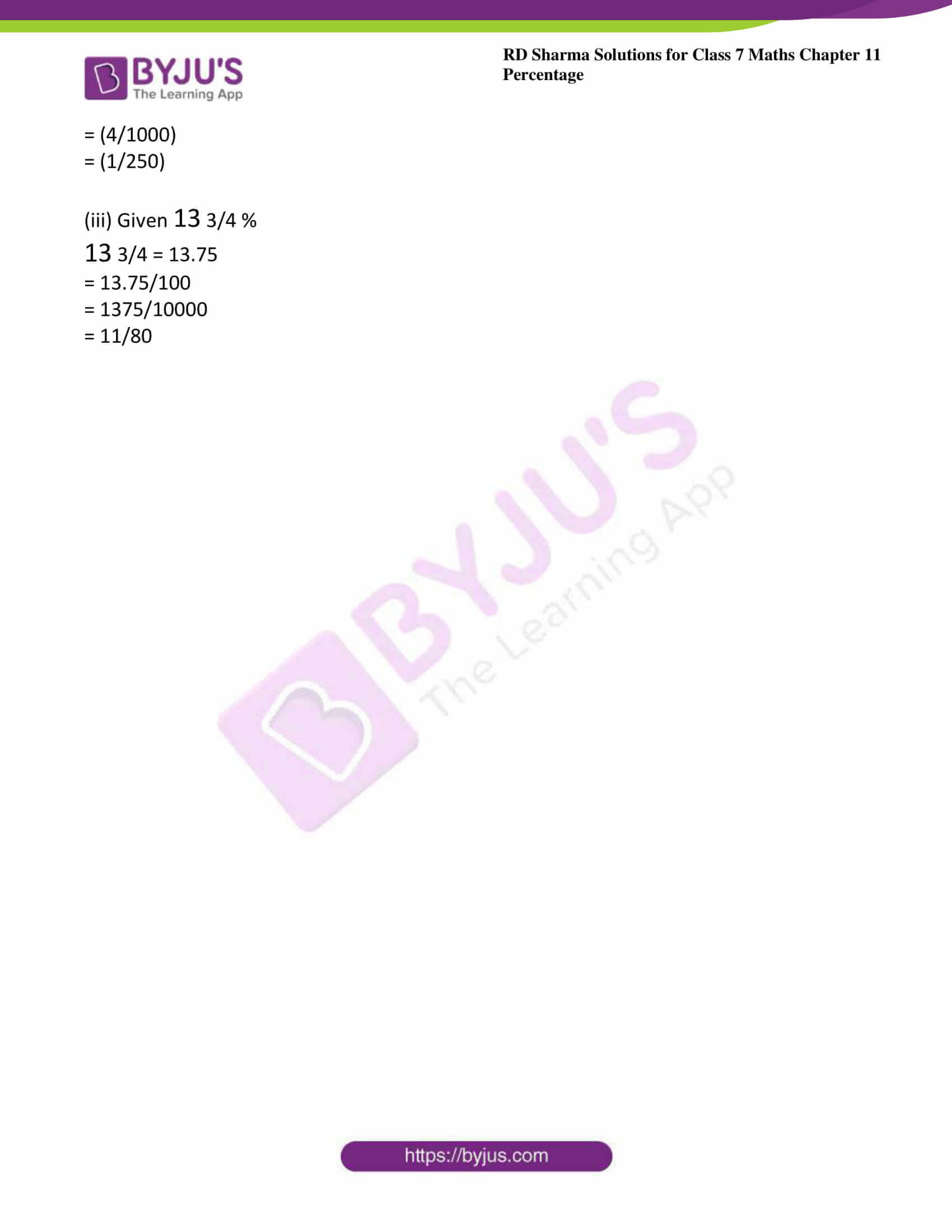# RD Sharma Solutions For Class 7 Maths Chapter 11 Percentage Exercise 11.2

The main objective of providing exercise-wise solutions in PDF is to help students speed up their exam preparation. The questions present in this exercise have been solved by BYJU’S experts in Maths, and these solutions guide students to study effectively. The students can download solutions which are available in PDF format easily. RD Sharma Solutions for Class 7 Maths Chapter 11 Percentage Exercise 11.2 are provided here. This exercise contains the step-wise method to the conversion of a ratio into percent and vice versa.

## Download the PDF of RD Sharma Solutions For Class 7 Maths Chapter 11 – Percentage Exercise 11.2### Access answers to Maths RD Sharma Solutions For Class 7 Chapter 11 – Percentage Exercise 11.2

Exercise 11.2 Page No: 11.4

1. Express each of the following ratios as per cents:

(i) 4: 5

(ii) 1: 5

(iii) 11: 125

Solution:

(i) Given 4: 5

4: 5 can be written as (4/5)

= (4/5) × 100

= 80%

(ii) Given 1: 5

1: 5 can be written as (1/5)

= (1/5) × 100

= 20%

(iii) Given 11: 125

11: 125 can be written as (11/125)

= (11/125) × 100

= (44/5) %

2. Express each of the following percents as ratios in the simplest form:

(i) 2.5%

(ii) 0.4%

(iii) 13 3/4 %

Solution:

(i) Given 2.5%

= (2.5/100)

= (25/1000)

= (1/40)

(ii) Given 0.4%

= (0.4/100)

= (4/1000)

= (1/250)

(iii) Given 13 3/4 %

13 3/4 = 13.75

= 13.75/100

= 1375/10000

= 11/80